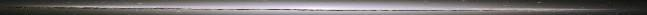# Unit Conversions

Please, enter numbers into any of the boxes below (the conversions work either way, from left to right and right to left).

To get the result, click in another box or anywhere else.

Special thanks to Carl Rod Nave (RodNave@gsu.edu) for this Unit Convertor written in 1998 (1st version, I changed the format much!). And also Joshua E. Barnes of the University of Hawaii for some of the astronimical conversions.

This convertor was taken from the Hyper Physics and Hyper Physics.

All the Physical Units.

You can also visit the Mechanics pages.

 meters ū 3.28407225 = feet centimeters ū .3937 = inches miles ū 5280 = feet miles ū 1609 = meters Newtons ū .2248 = pounds Newtons ū 100000 = dynes kilograms ū .06852 = slugs lb/in2 ū 6895 = Pascals lb/ft2 ū 47.88 = Pascals miles/hour ū 1.467 = ft/sec miles/hour ū 1.609 = km/hour meters/sec ū 2.24 = miles/hour km/hour ū 0.278 = meters/sec atmosphere ū 101.3 = kilopascals atmosphere ū 14.69 = lb/in2 lb/in2 ū 51.7 = mmHg mmHg ū 13.6 = mmH2O cmH2O ū 980 = dyne/cm2 mmHg ū 1333 = dyne/cm2 dynes/cm2 ū 0.1 = Pascals mmHg ū 133.3 = Pascals cmH2O ū 98 = Pascals cm3 ū .000001 = m3 in3 ū .0000163 = m3 in3 ū 16.39 = cm3 ft3 ū .02832 = m3 liters ū .001 = m3 gallons ū .003785 = m3 gallons ū 3785 = cm3 gallons ū 231 = in3 ft-lb ū 1.356 = joules ergs ū 0.1 = microjoule calories ū 4.186 = joules kilocalories ū 4186 = joules kilowatt hour ū 3.6 = Megajoules electron volt ū 1.6 = ū 10-19 joules acres ū 43560 = ft2 kg ū 2.2046226218487757 = pounds pounds „ 2000 = tons (non-metric) kg „ 907.2 = tons (non-metric) years ū 3.15 = ū 107 sec (seconds) AU (Astronomical Unit) ū 1.496 = ū 1011 m (meters) pc (Parsec) ū 2.062 = ū 105 AU pc (Parsec) ū 3.086 = ū 1016 AU Mū 5.967 = ū 1024 kg Mū 1.989 = ū 1030 kg hectare ū 10000 = square meters square kilometers ū 100 = hectare Celcius ū 1.8 + 32 = Fahrenheit

I found the following on the foldoc website...

# prefix

1. <unit> The standard metric prefixes used in the SystĶme International (SI) conventions for scientific measurement.

Here are the SI magnifying prefixes, along with the corresponding binary interpretations in common use:

``` prefix abr decimal  binary

yocto-     1000-8
zepto-     1000-7
atto-      1000-6
femto-  f  1000-5
pico-   p  1000-4
nano-   n  1000-3
micro-  *  1000-2          * Abbreviation: Greek mu (µ)
milli-  m  1000-1

kilo- 	 k  10001  10241 = 210 = 1,024
mega- 	 M  10002  10242 = 220 = 1,048,576
giga- 	 G  10003  10243 = 230 = 1,073,741,824
tera- 	 T  10004  10244 = 240 = 1,099,511,627,776
peta- 	    10005  10245 = 250 = 1,125,899,906,842,624
exa-  	    10006  10246 = 260 = 1,152,921,504,606,846,976
zetta-     10007  10247 = 270 = 1,180,591,620,717,411,303,424
yotta-     10008  10248 = 280 = 1,208,925,819,614,629,174,706,176

```
"Femto" and "atto" derive not from Greek but from Danish.

The abbreviated forms of these prefixes are common in electronics and physics.

When used with bytes of storage, these prefixes usually denote multiplication by powers of 1024 = 210 (K, M, and G are common in computing). Thus "MB" stands for megabytes (220 bytes). This common practice goes against the edicts of the BIPM who deprecate the use of these prefixes for powers of two. The formal SI prefix for 1000 is lower case "k"; some, including this dictionary, use this strictly, reserving upper case "K" for multiplication by 1024 (KB is thus "kilobytes").

Also, in data transfer rates the prefixes stand for powers of ten so, for example, 28.8 kb/s means 28,800 bits per second.

The unit is often dropped so one may talk of "a 40K salary" (40,000 dollars) or "2 meg of disk space" (2×220 bytes).

The accepted pronunciation of the initial G of "giga-" is hard, /gi'ga/.

Confusing 1000 and 1024 (or other powers of 2 and 10 close in magnitude) - for example, describing a memory in units of 500K or 524K instead of 512K - is a sure sign of the marketroid. For example, 3.5" microfloppies are often described as storing "1.44 MB". In fact, this is completely specious. The correct size is 1440 KB = 1440 × 1024 = 1474560 bytes. Alas, this point is probably lost on the world forever.

In 1993, hacker Morgan Burke proposed, to general approval on Usenet, the following additional prefixes: groucho (10-30), harpo (10-27), harpi (1027), grouchi (1030). This would leave the prefixes zeppo-, gummo-, and chico- available for future expansion. Sadly, there is little immediate prospect that Mr. Burke's eminently sensible proposal will be ratified.

2. <language> Related to the prefix notation.

(2003-05-06)Back to my home page.